Want to share your content on R-bloggers? click here if you have a blog, or here if you don't.

I would like to familiarize myself with machine learning (ML) techniques in `R`. So I have been reading and learning by doing. I thought I’d share my experience for others who’d like to give it a try1.

# First version August 13, 2021, updated August 23, 2021

Since my first post, I’ve been reading notebooks shared by folks who ranked high in the challenge, and added two features that they used. Eventually, these new predictors did not help (I must be doing something wrong). I also explored some other ML algorithms. Last, I tuned the parameters more efficiently with a clever grid-search algorithm. All in all, I slightly improved my score, but most importantly, I now have a clean template for further use.

# Motivation

All material available from GitHub at https://github.com/oliviergimenez/learning-machine-learning.

The two great books I’m using are:

I also recommend checking out the material (codes, screencasts) shared by David Robinson and Julia Silge from whom I picked some useful tricks that I put to use below.

To try things, I’ve joined the Kaggle online community which gathers folks with lots of experience in ML from whom you can learn. Kaggle also hosts public datasets that can be used for playing around.

I use the `tidymodels` metapackage that contains a suite of packages for modeling and machine learning using `tidyverse` principles. Check out all possibilities here, and parsnip models in particular there.

Let’s start with the famous Titanic dataset. We need to predict if a passenger survived the sinking of the Titanic (1) or not (0). A dataset is provided for training our models (train.csv). Another dataset is provided (test.csv) for which we do not know the answer. We will predict survival for each passenger, submit our answer to Kaggle and see how well we did compared to other folks. The metric for comparison is the percentage of passengers we correctly predict – aka as accuracy.

First things first, let’s load some packages to get us started.

```library(tidymodels) # metapackage for ML
library(tidyverse) # metapackage for data manipulation and visulaisation
library(stacks) # stack ML models for better perfomance
theme_set(theme_light())
doParallel::registerDoParallel(cores = 4) # parallel computations
```

# Data

```rawdata <- read_csv("dat/titanic/train.csv")
glimpse(rawdata)
## Rows: 891
## Columns: 12
## \$ PassengerId <dbl> 1, 2, 3, 4, 5, 6, 7, 8, 9, 10, 11, 12, 13, 14, 15, 16, 17, 18, 19, 20…
## \$ Survived <dbl> 0, 1, 1, 1, 0, 0, 0, 0, 1, 1, 1, 1, 0, 0, 0, 1, 0, 1, 0, 1, 0, 1, 1, …
## \$ Pclass <dbl> 3, 1, 3, 1, 3, 3, 1, 3, 3, 2, 3, 1, 3, 3, 3, 2, 3, 2, 3, 3, 2, 2, 3, …
## \$ Name <chr> "Braund, Mr. Owen Harris", "Cumings, Mrs. John Bradley (Florence Brig…
## \$ Sex <chr> "male", "female", "female", "female", "male", "male", "male", "male",…
## \$ Age <dbl> 22, 38, 26, 35, 35, NA, 54, 2, 27, 14, 4, 58, 20, 39, 14, 55, 2, NA, …
## \$ SibSp <dbl> 1, 1, 0, 1, 0, 0, 0, 3, 0, 1, 1, 0, 0, 1, 0, 0, 4, 0, 1, 0, 0, 0, 0, …
## \$ Parch <dbl> 0, 0, 0, 0, 0, 0, 0, 1, 2, 0, 1, 0, 0, 5, 0, 0, 1, 0, 0, 0, 0, 0, 0, …
## \$ Ticket <chr> "A/5 21171", "PC 17599", "STON/O2. 3101282", "113803", "373450", "330…
## \$ Fare <dbl> 7.2500, 71.2833, 7.9250, 53.1000, 8.0500, 8.4583, 51.8625, 21.0750, 1…
## \$ Cabin <chr> NA, "C85", NA, "C123", NA, NA, "E46", NA, NA, NA, "G6", "C103", NA, N…
## \$ Embarked <chr> "S", "C", "S", "S", "S", "Q", "S", "S", "S", "C", "S", "S", "S", "S",…
naniar::miss_var_summary(rawdata)
## # A tibble: 12 × 3
## variable n_miss pct_miss
## <chr> <int> <dbl>
## 1 Cabin 687 77.1
## 2 Age 177 19.9
## 3 Embarked 2 0.224
## 4 PassengerId 0 0
## 5 Survived 0 0
## 6 Pclass 0 0
## 7 Name 0 0
## 8 Sex 0 0
## 9 SibSp 0 0
## 10 Parch 0 0
## 11 Ticket 0 0
## 12 Fare 0 0
```

After some data exploration (not shown), I decided to take care of missing values, gather the two family variables in a single variable, and create a variable title.

```# Get most frequent port of embarkation
uniqx <- unique(na.omit(rawdata\$Embarked))
mode_embarked <- as.character(fct_drop(uniqx[which.max(tabulate(match(rawdata\$Embarked, uniqx)))]))
# Build function for data cleaning and handling NAs
process_data <- function(tbl){
tbl %>%
mutate(class = case_when(Pclass == 1 ~ "first",
Pclass == 2 ~ "second",
Pclass == 3 ~ "third"),
class = as_factor(class),
gender = factor(Sex),
fare = Fare,
age = Age,
ticket = Ticket,
alone = if_else(SibSp + Parch == 0, "yes", "no"), # alone variable
alone = as_factor(alone),
port = factor(Embarked), # rename embarked as port
title = str_extract(Name, "[A-Za-z]+\\."), # title variable
title = fct_lump(title, 4)) %>% # keep only most frequent levels of title
mutate(port = ifelse(is.na(port), mode_embarked, port), # deal w/ NAs in port (replace by mode)
port = as_factor(port)) %>%
group_by(title) %>%
mutate(median_age_title = median(age, na.rm = T)) %>%
ungroup() %>%
mutate(age = if_else(is.na(age), median_age_title, age)) %>% # deal w/ NAs in age (replace by median in title)
mutate(ticketfreq = ave(1:nrow(.), FUN = length),
fareadjusted = fare / ticketfreq) %>%
mutate(familyage = SibSp + Parch + 1 + age/70)
}
# Process the data
dataset <- rawdata %>%
process_data() %>%
mutate(survived = as_factor(if_else(Survived == 1, "yes", "no"))) %>%
mutate(survived = relevel(survived, ref = "yes")) %>% # first event is survived = yes
select(survived, class, gender, age, alone, port, title, fareadjusted, familyage)
# Have a look again
glimpse(dataset)
## Rows: 891
## Columns: 9
## \$ survived <fct> no, yes, yes, yes, no, no, no, no, yes, yes, yes, yes, no, no, no, y…
## \$ class <fct> third, first, third, first, third, third, first, third, third, secon…
## \$ gender <fct> male, female, female, female, male, male, male, male, female, female…
## \$ age <dbl> 22, 38, 26, 35, 35, 30, 54, 2, 27, 14, 4, 58, 20, 39, 14, 55, 2, 30,…
## \$ alone <fct> no, no, yes, no, yes, yes, yes, no, no, no, no, yes, yes, no, yes, y…
## \$ port <fct> 3, 1, 3, 3, 3, 2, 3, 3, 3, 1, 3, 3, 3, 3, 3, 3, 2, 3, 3, 1, 3, 3, 2,…
## \$ title <fct> Mr., Mrs., Miss., Mrs., Mr., Mr., Mr., Master., Mrs., Mrs., Miss., M…
## \$ fareadjusted <dbl> 0.008136925, 0.080003704, 0.008894501, 0.059595960, 0.009034792, 0.0…
## \$ familyage <dbl> 2.314286, 2.542857, 1.371429, 2.500000, 1.500000, 1.428571, 1.771429…
naniar::miss_var_summary(dataset)
## # A tibble: 9 × 3
## variable n_miss pct_miss
## <chr> <int> <dbl>
## 1 survived 0 0
## 2 class 0 0
## 3 gender 0 0
## 4 age 0 0
## 5 alone 0 0
## 6 port 0 0
## 7 title 0 0
## 9 familyage 0 0
```

Let’s apply the same treatment to the test dataset.

```rawdata <- read_csv("dat/titanic/test.csv")
holdout <- rawdata %>%
process_data() %>%
select(PassengerId, class, gender, age, alone, port, title, fareadjusted, familyage)
glimpse(holdout)
## Rows: 418
## Columns: 9
## \$ PassengerId <dbl> 892, 893, 894, 895, 896, 897, 898, 899, 900, 901, 902, 903, 904, 905…
## \$ class <fct> third, third, second, third, third, third, third, second, third, thi…
## \$ gender <fct> male, female, male, male, female, male, female, male, female, male, …
## \$ age <dbl> 34.5, 47.0, 62.0, 27.0, 22.0, 14.0, 30.0, 26.0, 18.0, 21.0, 28.5, 46…
## \$ alone <fct> yes, no, yes, yes, no, yes, yes, no, yes, no, yes, yes, no, no, no, …
## \$ port <fct> 2, 3, 2, 3, 3, 3, 2, 3, 1, 3, 3, 3, 3, 3, 3, 1, 2, 1, 3, 1, 1, 3, 3,…
## \$ title <fct> Mr., Mrs., Mr., Mr., Mrs., Mr., Miss., Mr., Mrs., Mr., Mr., Mr., Mrs…
## \$ fareadjusted <dbl> 0.018730144, 0.016746411, 0.023175837, 0.020723684, 0.029395933, 0.0…
## \$ familyage <dbl> 1.492857, 2.671429, 1.885714, 1.385714, 3.314286, 1.200000, 1.428571…
naniar::miss_var_summary(holdout)
## # A tibble: 9 × 3
## variable n_miss pct_miss
## <chr> <int> <dbl>
## 2 PassengerId 0 0
## 3 class 0 0
## 4 gender 0 0
## 5 age 0 0
## 6 alone 0 0
## 7 port 0 0
## 8 title 0 0
## 9 familyage 0 0
```

# Exploratory data analysis

```skimr::skim(dataset)
```
 Name dataset Number of rows 891 Number of columns 9 _______________________ Column type frequency: factor 6 numeric 3 ________________________ Group variables None

Data summary

Variable type: factor

skim_variable n_missing complete_rate ordered n_unique top_counts
survived 0 1 FALSE 2 no: 549, yes: 342
class 0 1 FALSE 3 thi: 491, fir: 216, sec: 184
gender 0 1 FALSE 2 mal: 577, fem: 314
alone 0 1 FALSE 2 yes: 537, no: 354
port 0 1 FALSE 4 3: 644, 1: 168, 2: 77, S: 2
title 0 1 FALSE 5 Mr.: 517, Mis: 182, Mrs: 125, Mas: 40

Variable type: numeric

skim_variable n_missing complete_rate mean sd p0 p25 p50 p75 p100 hist
age 0 1 29.39 13.26 0.42 21.00 30.00 35.00 80.00 ▂▇▃▁▁
fareadjusted 0 1 0.04 0.06 0.00 0.01 0.02 0.03 0.58 ▇▁▁▁▁
familyage 0 1 2.32 1.57 1.07 1.41 1.57 2.62 11.43 ▇▁▁▁▁

Let’s explore the data.

```dataset %>%
count(survived)
## # A tibble: 2 × 2
## survived n
## <fct> <int>
## 1 yes 342
## 2 no 549
dataset %>%
group_by(gender) %>%
summarize(n = n(),
n_surv = sum(survived == "yes"),
pct_surv = n_surv / n)
## # A tibble: 2 × 4
## gender n n_surv pct_surv
## <fct> <int> <int> <dbl>
## 1 female 314 233 0.742
## 2 male 577 109 0.189
dataset %>%
group_by(title) %>%
summarize(n = n(),
n_surv = sum(survived == "yes"),
pct_surv = n_surv / n) %>%
arrange(desc(pct_surv))
## # A tibble: 5 × 4
## title n n_surv pct_surv
## <fct> <int> <int> <dbl>
## 1 Mrs. 125 99 0.792
## 2 Miss. 182 127 0.698
## 3 Master. 40 23 0.575
## 4 Other 27 12 0.444
## 5 Mr. 517 81 0.157
dataset %>%
group_by(class, gender) %>%
summarize(n = n(),
n_surv = sum(survived == "yes"),
pct_surv = n_surv / n) %>%
arrange(desc(pct_surv))
## # A tibble: 6 × 5
## # Groups: class 
## class gender n n_surv pct_surv
## <fct> <fct> <int> <int> <dbl>
## 1 first female 94 91 0.968
## 2 second female 76 70 0.921
## 3 third female 144 72 0.5
## 4 first male 122 45 0.369
## 5 second male 108 17 0.157
## 6 third male 347 47 0.135
```

Some informative graphs.

```dataset %>%
group_by(class, gender) %>%
summarize(n = n(),
n_surv = sum(survived == "yes"),
pct_surv = n_surv / n) %>%
mutate(class = fct_reorder(class, pct_surv)) %>%
ggplot(aes(pct_surv, class, fill = class, color = class)) +
geom_col(position = position_dodge()) +
scale_x_continuous(labels = percent) +
labs(x = "% in category that survived", fill = NULL, color = NULL, y = NULL) +
facet_wrap(~gender)
``````dataset %>%
mutate(age = cut(age, breaks = c(0, 20, 40, 60, 80))) %>%
group_by(age, gender) %>%
summarize(n = n(),
n_surv = sum(survived == "yes"),
pct_surv = n_surv / n) %>%
mutate(age = fct_reorder(age, pct_surv)) %>%
ggplot(aes(pct_surv, age, fill = age, color = age)) +
geom_col(position = position_dodge()) +
scale_x_continuous(labels = percent) +
labs(x = "% in category that survived", fill = NULL, color = NULL, y = NULL) +
facet_wrap(~gender)
``````dataset %>%
ggplot(aes(fareadjusted, group = survived, color = survived, fill = survived)) +
geom_histogram(alpha = .4, position = position_dodge()) +
labs(x = "fare", y = NULL, color = "survived?", fill = "survived?")
``````dataset %>%
ggplot(aes(familyage, group = survived, color = survived, fill = survived)) +
geom_histogram(alpha = .4, position = position_dodge()) +
labs(x = "family aged", y = NULL, color = "survived?", fill = "survived?")
```# Training/testing datasets

Split our dataset in two, one dataset for training and the other one for testing. We will use an additionnal splitting step for cross-validation.

```set.seed(2021)
spl <- initial_split(dataset, strata = "survived")
train <- training(spl)
test <- testing(spl)
train_5fold <- train %>%
vfold_cv(5)
```

# Gradient boosting algorithms – xgboost

## Tuning

Set up defaults.

```mset <- metric_set(accuracy) # metric is accuracy
control <- control_grid(save_workflow = TRUE,
save_pred = TRUE,
extract = extract_model) # grid for tuning
```

First a recipe.

```xg_rec <- recipe(survived ~ ., data = train) %>%
step_impute_median(all_numeric()) %>% # replace missing value by median
step_dummy(all_nominal_predictors()) # all factors var are split into binary terms (factor disj coding)
```

Then specify a gradient boosting model.

```xg_model <- boost_tree(mode = "classification", # binary response
trees = tune(),
mtry = tune(),
tree_depth = tune(),
learn_rate = tune(),
loss_reduction = tune(),
min_n = tune()) # parameters to be tuned
```

Now set our workflow.

```xg_wf <-
workflow() %>%
```

Use cross-validation to evaluate our model with different param config.

```xg_tune <- xg_wf %>%
tune_grid(train_5fold,
metrics = mset,
control = control,
grid = crossing(trees = 1000,
mtry = c(3, 5, 8), # finalize(mtry(), train)
tree_depth = c(5, 10, 15),
learn_rate = c(0.01, 0.005),
loss_reduction = c(0.01, 0.1, 1),
min_n = c(2, 10, 25)))
```

Visualize the results.

```autoplot(xg_tune) + theme_light()
```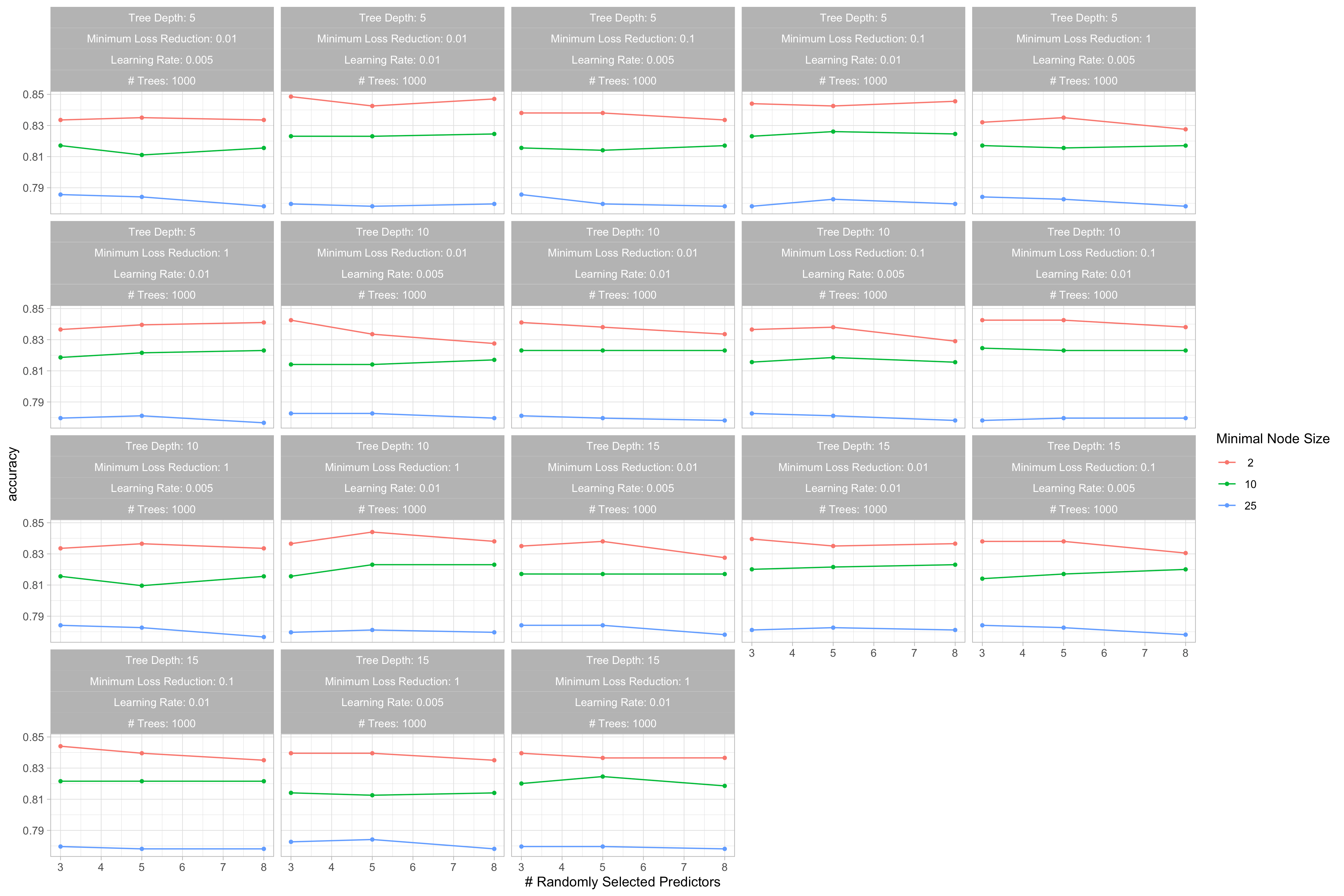Collect metrics.

```xg_tune %>%
collect_metrics() %>%
arrange(desc(mean))
## # A tibble: 162 × 12
## mtry trees min_n tree_depth learn_rate loss_reduction .metric .estimator mean n
## <dbl> <dbl> <dbl> <dbl> <dbl> <dbl> <chr> <chr> <dbl> <int>
## 1 3 1000 2 5 0.01 0.01 accuracy binary 0.849 5
## 2 8 1000 2 5 0.01 0.01 accuracy binary 0.847 5
## 3 8 1000 2 5 0.01 0.1 accuracy binary 0.846 5
## 4 3 1000 2 15 0.01 0.1 accuracy binary 0.844 5
## 5 5 1000 2 10 0.01 1 accuracy binary 0.844 5
## 6 3 1000 2 5 0.01 0.1 accuracy binary 0.844 5
## 7 5 1000 2 10 0.01 0.1 accuracy binary 0.843 5
## 8 3 1000 2 10 0.01 0.1 accuracy binary 0.843 5
## 9 5 1000 2 5 0.01 0.01 accuracy binary 0.843 5
## 10 5 1000 2 5 0.01 0.1 accuracy binary 0.843 5
## # … with 152 more rows, and 2 more variables: std_err <dbl>, .config <chr>
```

The tuning takes some time. There are other ways to explore the parameter space more efficiently. For example, we will use the function `dials::grid_max_entropy()` in the last section about ensemble modelling. Here, I will use `finetune::tune_race_anova`.

```library(finetune)
xg_tune <-
xg_wf %>%
tune_race_anova(
train_5fold,
grid = 50,
param_info = xg_model %>% parameters(),
metrics = metric_set(accuracy),
control = control_race(verbose_elim = TRUE))
```

Visualize the results.

```autoplot(xg_tune)
```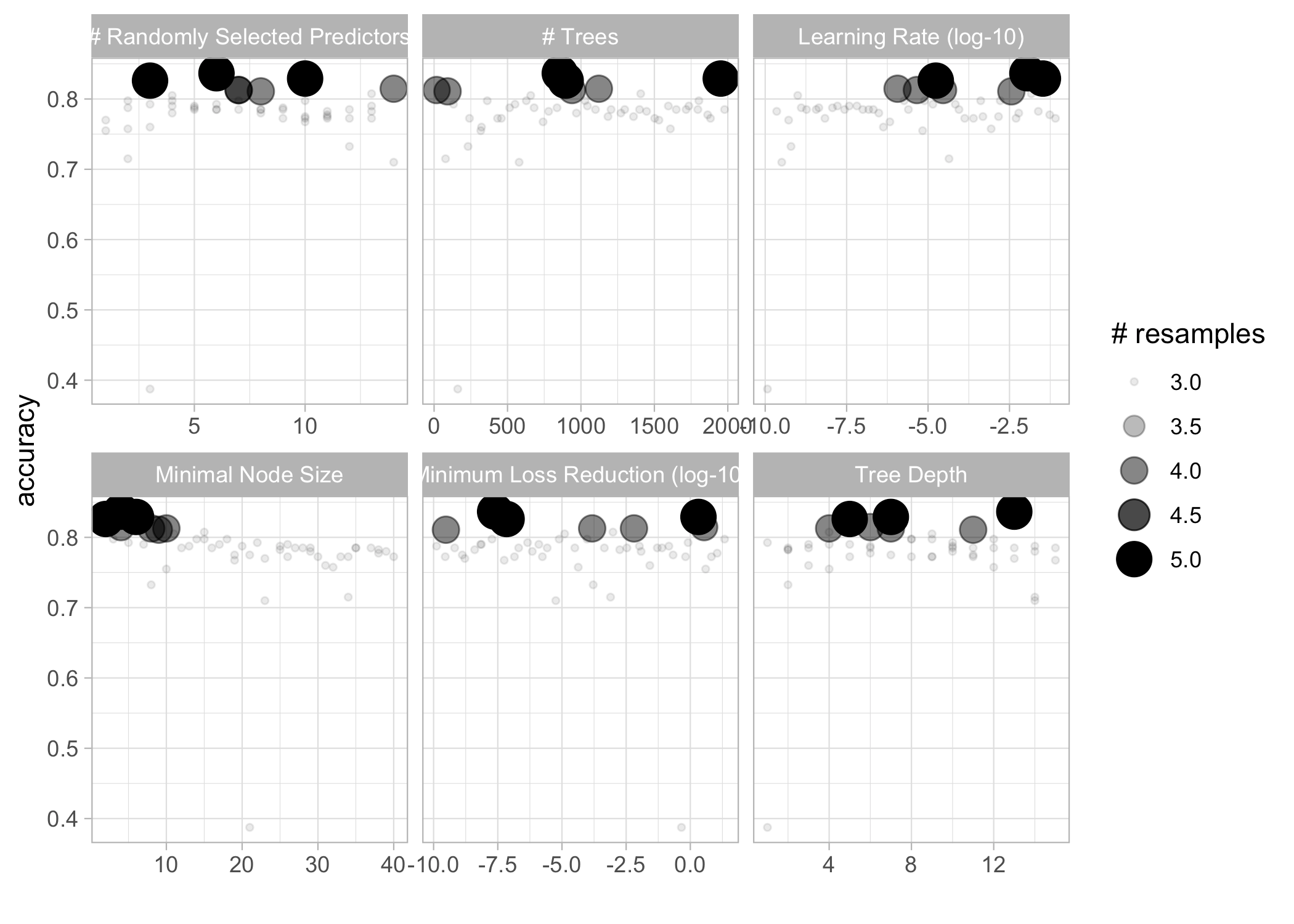Collect metrics.

```xg_tune %>%
collect_metrics() %>%
arrange(desc(mean))
## # A tibble: 50 × 12
## mtry trees min_n tree_depth learn_rate loss_reduction .metric .estimator mean n
## <int> <int> <int> <int> <dbl> <dbl> <chr> <chr> <dbl> <int>
## 1 6 856 4 13 1.12e- 2 2.49e- 8 accuracy binary 0.837 5
## 2 10 1952 6 7 3.36e- 2 2.07e+ 0 accuracy binary 0.829 5
## 3 3 896 2 5 1.73e- 5 6.97e- 8 accuracy binary 0.826 5
## 4 14 1122 4 6 1.16e- 6 3.44e+ 0 accuracy binary 0.815 4
## 5 7 939 8 4 2.88e- 5 1.50e- 4 accuracy binary 0.813 4
## 6 7 17 10 7 4.54e- 6 6.38e- 3 accuracy binary 0.813 4
## 7 8 92 9 11 3.60e- 3 3.01e-10 accuracy binary 0.811 4
## 8 13 1407 15 4 1.48e- 2 9.68e- 4 accuracy binary 0.807 3
## 9 4 658 11 9 9.97e-10 1.27e- 5 accuracy binary 0.805 3
## 10 2 628 14 9 1.84e- 6 9.44e- 5 accuracy binary 0.798 3
## # … with 40 more rows, and 2 more variables: std_err <dbl>, .config <chr>
```

## Fit model

Use best config to fit model to training data.

```xg_fit <- xg_wf %>%
finalize_workflow(select_best(xg_tune)) %>%
fit(train)
## [23:39:25] WARNING: amalgamation/../src/learner.cc:1095: Starting in XGBoost 1.3.0, the default evaluation metric used with the objective 'binary:logistic' was changed from 'error' to 'logloss'. Explicitly set eval_metric if you'd like to restore the old behavior.
```

Check out accuracy on testing dataset to see if we overfitted.

```xg_fit %>%
augment(test, type.predict = "response") %>%
accuracy(survived, .pred_class)
## # A tibble: 1 × 3
## .metric .estimator .estimate
## <chr> <chr> <dbl>
## 1 accuracy binary 0.812
```

Check out important features (aka predictors).

```importances <- xgboost::xgb.importance(model = extract_fit_engine(xg_fit))
importances %>%
mutate(Feature = fct_reorder(Feature, Gain)) %>%
ggplot(aes(Gain, Feature)) +
geom_col()
```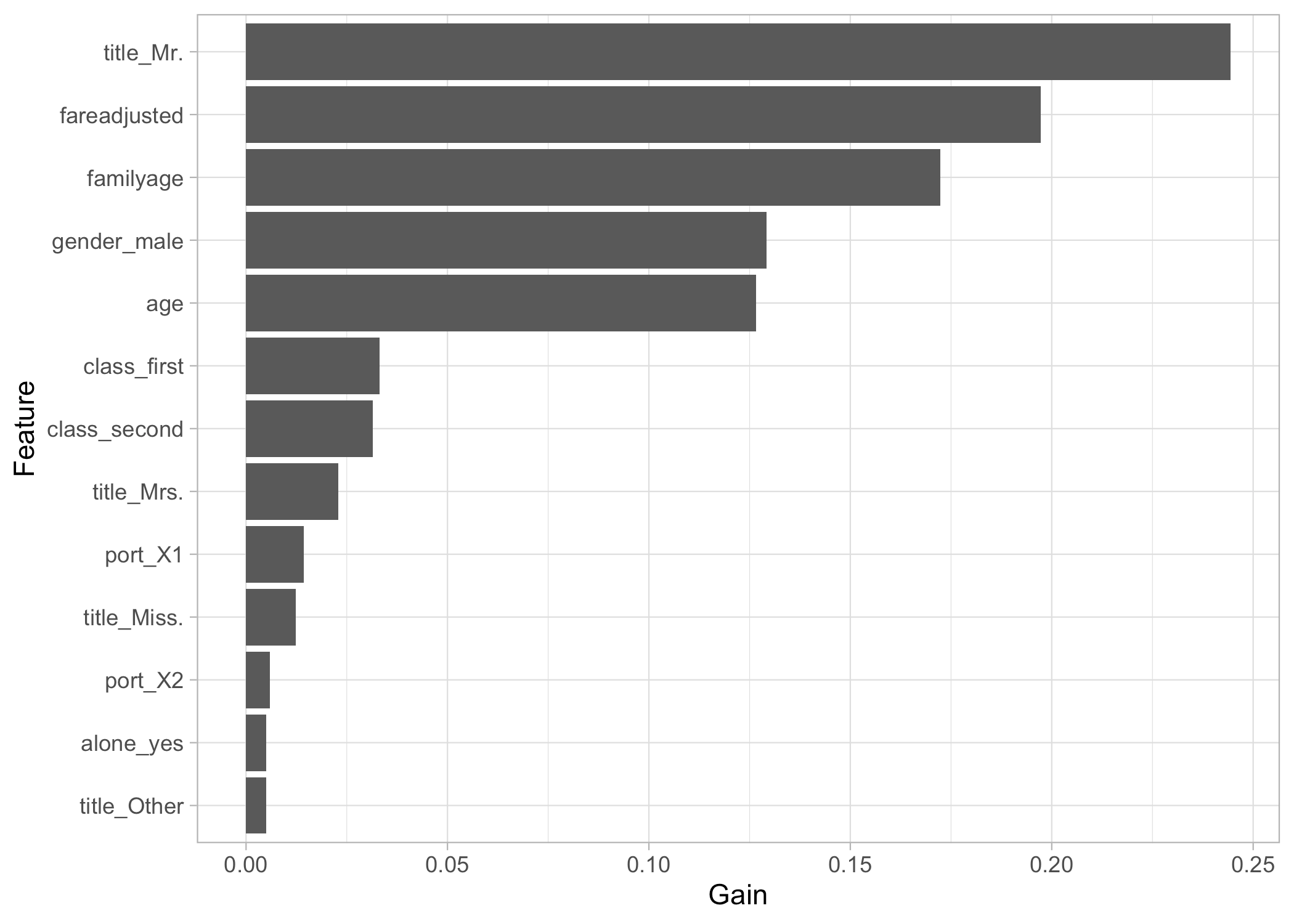## Make predictions

Now we’re ready to predict survival for the holdout dataset and submit to Kaggle. Note that I use the whole dataset, not just the training dataset.

```xg_wf %>%
finalize_workflow(select_best(xg_tune)) %>%
fit(dataset) %>%
augment(holdout) %>%
select(PassengerId, Survived = .pred_class) %>%
mutate(Survived = if_else(Survived == "yes", 1, 0)) %>%
write_csv("output/titanic/xgboost.csv")
## [23:39:28] WARNING: amalgamation/../src/learner.cc:1095: Starting in XGBoost 1.3.0, the default evaluation metric used with the objective 'binary:logistic' was changed from 'error' to 'logloss'. Explicitly set eval_metric if you'd like to restore the old behavior.
```

I got and accuracy of 0.74162. Cool. Let’s train a random forest model now.

# Random forests

Let’s continue with random forest methods.

## Tuning

First a recipe.

```rf_rec <- recipe(survived ~ ., data = train) %>%
step_impute_median(all_numeric()) %>% # replace missing value by median
step_dummy(all_nominal_predictors()) # all factors var are split into binary terms (factor disj coding)
```

Then specify a random forest model.

```rf_model <- rand_forest(mode = "classification", # binary response
engine = "ranger", # by default
mtry = tune(),
trees = tune(),
min_n = tune()) # parameters to be tuned
```

Now set our workflow.

```rf_wf <-
workflow() %>%
```

Use cross-validation to evaluate our model with different param config.

```rf_tune <-
rf_wf %>%
tune_race_anova(
train_5fold,
grid = 50,
param_info = rf_model %>% parameters(),
metrics = metric_set(accuracy),
control = control_race(verbose_elim = TRUE))
```

Visualize the results.

```autoplot(rf_tune)
```Collect metrics.

```rf_tune %>%
collect_metrics() %>%
arrange(desc(mean))
## # A tibble: 50 × 9
## mtry trees min_n .metric .estimator mean n std_err .config
## <int> <int> <int> <chr> <chr> <dbl> <int> <dbl> <chr>
## 1 14 1554 5 accuracy binary 0.837 5 0.00656 Preprocessor1_Model49
## 2 4 774 18 accuracy binary 0.837 5 0.0133 Preprocessor1_Model50
## 3 5 1736 8 accuracy binary 0.834 5 0.0111 Preprocessor1_Model46
## 4 8 1322 5 accuracy binary 0.832 5 0.00713 Preprocessor1_Model41
## 5 2 1078 30 accuracy binary 0.831 5 0.00727 Preprocessor1_Model12
## 6 4 1892 14 accuracy binary 0.831 5 0.00886 Preprocessor1_Model39
## 7 8 962 7 accuracy binary 0.829 5 0.00742 Preprocessor1_Model43
## 8 7 946 4 accuracy binary 0.826 5 0.00452 Preprocessor1_Model23
## 9 7 1262 3 accuracy binary 0.826 5 0.00710 Preprocessor1_Model47
## 10 9 544 9 accuracy binary 0.825 5 0.00673 Preprocessor1_Model11
## # … with 40 more rows
```

## Fit model

Use best config to fit model to training data.

```rf_fit <- rf_wf %>%
finalize_workflow(select_best(rf_tune)) %>%
fit(train)
```

Check out accuracy on testing dataset to see if we overfitted.

```rf_fit %>%
augment(test, type.predict = "response") %>%
accuracy(survived, .pred_class)
## # A tibble: 1 × 3
## .metric .estimator .estimate
## <chr> <chr> <dbl>
## 1 accuracy binary 0.786
```

Check out important features (aka predictors).

```library(vip)
finalize_model(
x = rf_model,
parameters = select_best(rf_tune)) %>%
set_engine("ranger", importance = "permutation") %>%
fit(survived ~ ., data = juice(prep(rf_rec))) %>%
vip(geom = "point")
```## Make predictions

Now we’re ready to predict survival for the holdout dataset and submit to Kaggle.

```rf_wf %>%
finalize_workflow(select_best(rf_tune)) %>%
fit(dataset) %>%
augment(holdout) %>%
select(PassengerId, Survived = .pred_class) %>%
mutate(Survived = if_else(Survived == "yes", 1, 0)) %>%
write_csv("output/titanic/randomforest.csv")
```

I got and accuracy of 0.77990, a bit better than gradient boosting.

Let’s continue with cat boosting methods.

# Gradient boosting algorithms - catboost

## Tuning

Set up defaults.

```mset <- metric_set(accuracy) # metric is accuracy
control <- control_grid(save_workflow = TRUE,
save_pred = TRUE,
extract = extract_model) # grid for tuning
```

First a recipe.

```cb_rec <- recipe(survived ~ ., data = train) %>%
step_impute_median(all_numeric()) %>% # replace missing value by median
step_dummy(all_nominal_predictors()) # all factors var are split into binary terms (factor disj coding)
```

Then specify a cat boosting model.

```library(treesnip)
cb_model <- boost_tree(mode = "classification",
engine = "catboost",
mtry = tune(),
trees = tune(),
min_n = tune(),
tree_depth = tune(),
learn_rate = tune()) # parameters to be tuned
```

Now set our workflow.

```cb_wf <-
workflow() %>%
```

Use cross-validation to evaluate our model with different param config.

```cb_tune <- cb_wf %>%
tune_race_anova(
train_5fold,
grid = 30,
param_info = cb_model %>% parameters(),
metrics = metric_set(accuracy),
control = control_race(verbose_elim = TRUE))
```

Visualize the results.

```autoplot(cb_tune)
```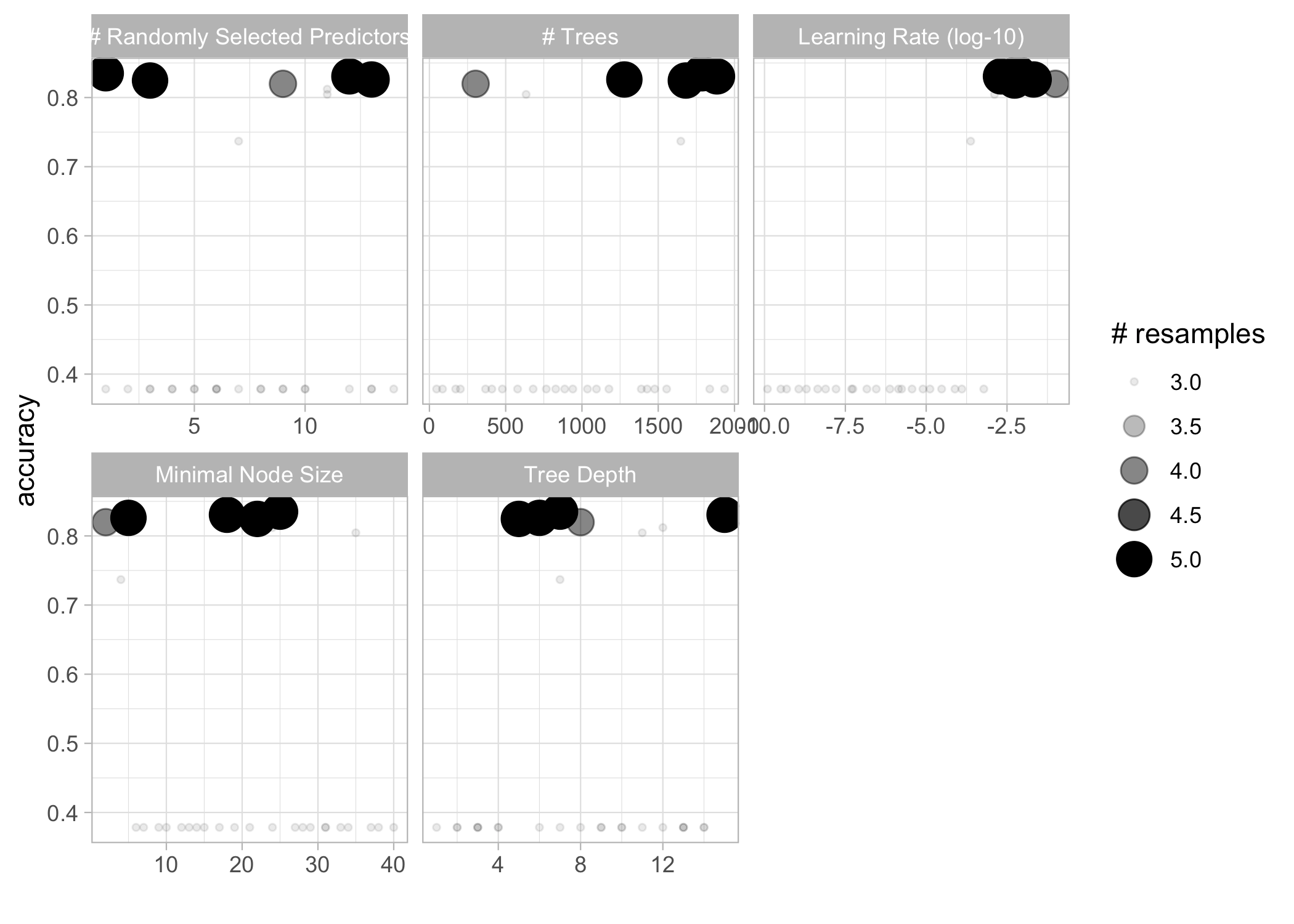Collect metrics.

```cb_tune %>%
collect_metrics() %>%
arrange(desc(mean))
## # A tibble: 30 × 11
## mtry trees min_n tree_depth learn_rate .metric .estimator mean n std_err .config
## <int> <int> <int> <int> <dbl> <chr> <chr> <dbl> <int> <dbl> <chr>
## 1 1 1787 25 7 6.84e- 3 accuracy binary 0.835 5 0.0125 Preproc…
## 2 12 1885 18 15 2.06e- 3 accuracy binary 0.831 5 0.00602 Preproc…
## 3 13 1278 5 6 2.10e- 2 accuracy binary 0.826 5 0.00431 Preproc…
## 4 3 1681 22 5 5.42e- 3 accuracy binary 0.825 5 0.00507 Preproc…
## 5 9 303 2 8 9.94e- 2 accuracy binary 0.820 4 0.0120 Preproc…
## 6 11 1201 24 12 3.77e- 2 accuracy binary 0.812 3 0.00868 Preproc…
## 7 11 634 35 11 1.30e- 3 accuracy binary 0.805 3 0.0242 Preproc…
## 8 7 1648 4 7 2.38e- 4 accuracy binary 0.737 3 0.0115 Preproc…
## 9 1 1427 27 10 1.74e- 6 accuracy binary 0.378 3 0.0152 Preproc…
## 10 2 940 19 3 3.20e-10 accuracy binary 0.378 3 0.0152 Preproc…
## # … with 20 more rows
```

## Fit model

Use best config to fit model to training data.

```cb_fit <- cb_wf %>%
finalize_workflow(select_best(cb_tune)) %>%
fit(train)
```

Check out accuracy on testing dataset to see if we overfitted.

```cb_fit %>%
augment(test, type.predict = "response") %>%
accuracy(survived, .pred_class)
## # A tibble: 1 × 3
## .metric .estimator .estimate
## <chr> <chr> <dbl>
## 1 accuracy binary 0.808
```

## Make predictions

Now we’re ready to predict survival for the holdout dataset and submit to Kaggle.

```cb_wf %>%
finalize_workflow(select_best(cb_tune)) %>%
fit(dataset) %>%
augment(holdout) %>%
select(PassengerId, Survived = .pred_class) %>%
mutate(Survived = if_else(Survived == "yes", 1, 0)) %>%
write_csv("output/titanic/catboost.csv")
```

I got and accuracy of 0.76076. Cool.

# Regularization methods

Let’s continue with elastic net regularization.

## Tuning

First a recipe.

```en_rec <- recipe(survived ~ ., data = train) %>%
step_impute_median(all_numeric()) %>% # replace missing value by median
step_normalize(all_numeric_predictors()) %>% # normalize
step_dummy(all_nominal_predictors()) # all factors var are split into binary terms (factor disj coding)
```

Then specify a regularization model. We tune parameter mixture, with ridge regression for mixture = 0, and lasso for mixture = 1.

```en_model <- logistic_reg(penalty = tune(),
mixture = tune()) %>% # param to be tuned
set_engine("glmnet") %>% # elastic net
set_mode("classification") # binary response
```

Now set our workflow.

```en_wf <-
workflow() %>%
```

Use cross-validation to evaluate our model with different param config.

```en_tune <- en_wf %>%
tune_grid(train_5fold,
metrics = mset,
control = control,
grid = crossing(penalty = 10 ^ seq(-8, -.5, .5),
mixture = seq(0, 1, length.out = 10)))
```

Visualize the results.

```autoplot(en_tune)
```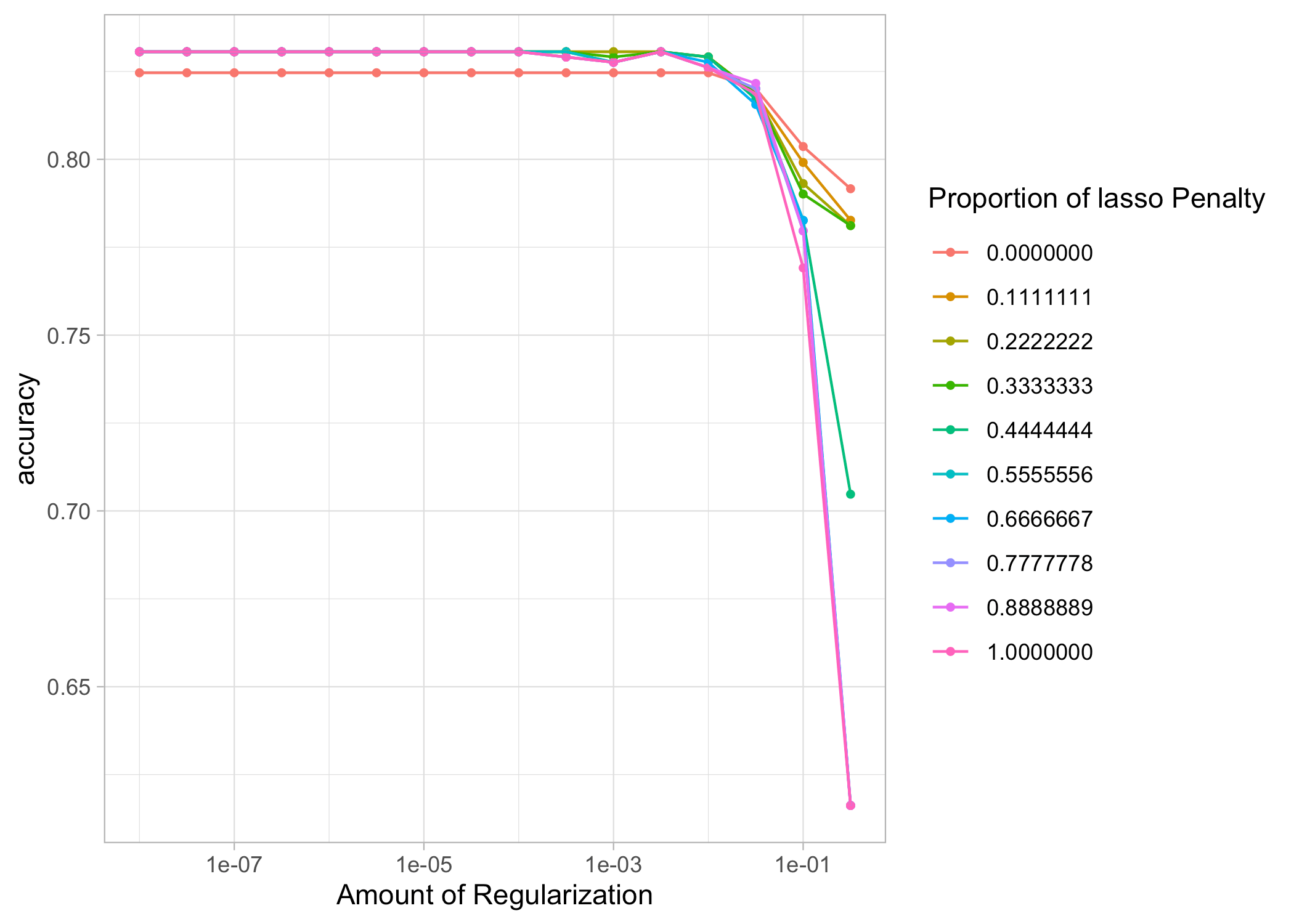Collect metrics.

```en_tune %>%
collect_metrics() %>%
arrange(desc(mean))
## # A tibble: 160 × 8
## penalty mixture .metric .estimator mean n std_err .config
## <dbl> <dbl> <chr> <chr> <dbl> <int> <dbl> <chr>
## 1 0.00000001 0.111 accuracy binary 0.831 5 0.0112 Preprocessor1_Model017
## 2 0.0000000316 0.111 accuracy binary 0.831 5 0.0112 Preprocessor1_Model018
## 3 0.0000001 0.111 accuracy binary 0.831 5 0.0112 Preprocessor1_Model019
## 4 0.000000316 0.111 accuracy binary 0.831 5 0.0112 Preprocessor1_Model020
## 5 0.000001 0.111 accuracy binary 0.831 5 0.0112 Preprocessor1_Model021
## 6 0.00000316 0.111 accuracy binary 0.831 5 0.0112 Preprocessor1_Model022
## 7 0.00001 0.111 accuracy binary 0.831 5 0.0112 Preprocessor1_Model023
## 8 0.0000316 0.111 accuracy binary 0.831 5 0.0112 Preprocessor1_Model024
## 9 0.0001 0.111 accuracy binary 0.831 5 0.0112 Preprocessor1_Model025
## 10 0.000316 0.111 accuracy binary 0.831 5 0.0112 Preprocessor1_Model026
## # … with 150 more rows
```

## Fit model

Use best config to fit model to training data.

```en_fit <- en_wf %>%
finalize_workflow(select_best(en_tune)) %>%
fit(train)
```

Check out accuracy on testing dataset to see if we overfitted.

```en_fit %>%
augment(test, type.predict = "response") %>%
accuracy(survived, .pred_class)
## # A tibble: 1 × 3
## .metric .estimator .estimate
## <chr> <chr> <dbl>
## 1 accuracy binary 0.826
```

Check out important features (aka predictors).

```library(broom)
en_fit\$fit\$fit\$fit %>%
tidy() %>%
filter(lambda >= select_best(en_tune)\$penalty) %>%
filter(lambda == min(lambda),
term != "(Intercept)") %>%
mutate(term = fct_reorder(term, estimate)) %>%
ggplot(aes(estimate, term, fill = estimate > 0)) +
geom_col() +
theme(legend.position = "none")
```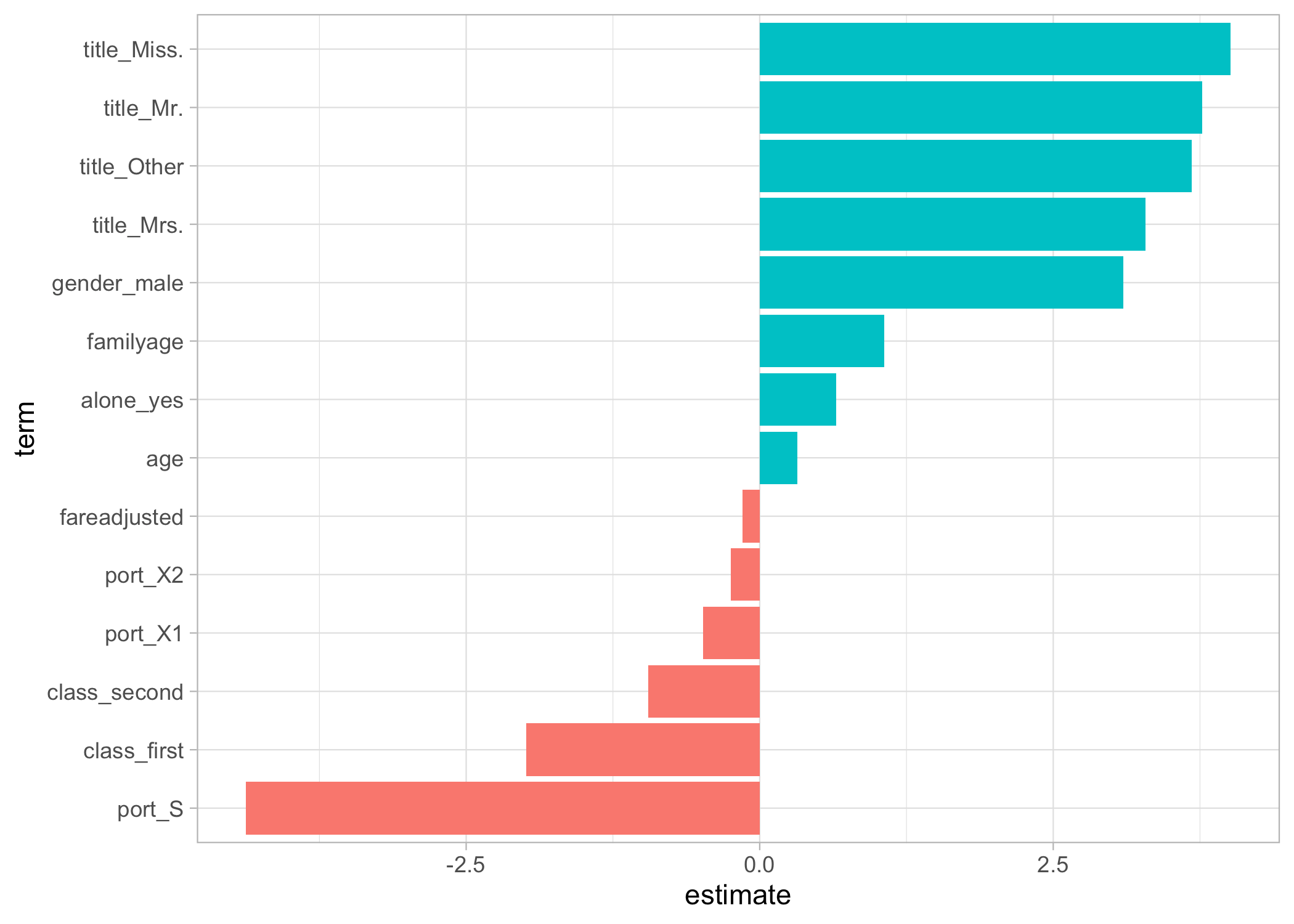## Make predictions

Now we’re ready to predict survival for the holdout dataset and submit to Kaggle.

```en_wf %>%
finalize_workflow(select_best(en_tune)) %>%
fit(dataset) %>%
augment(holdout) %>%
select(PassengerId, Survived = .pred_class) %>%
mutate(Survived = if_else(Survived == "yes", 1, 0)) %>%
write_csv("output/titanic/regularization.csv")
```

I got and accuracy of 0.76315.

# Logistic regression

And what about a good old-fashioned logistic regression (not a ML algo)?

First a recipe.

```logistic_rec <- recipe(survived ~ ., data = train) %>%
step_impute_median(all_numeric()) %>% # replace missing value by median
step_normalize(all_numeric_predictors()) %>% # normalize
step_dummy(all_nominal_predictors()) # all factors var are split into binary terms (factor disj coding)
```

Then specify a logistic regression.

```logistic_model <- logistic_reg() %>% # no param to be tuned
set_engine("glm") %>% # elastic net
set_mode("classification") # binary response
```

Now set our workflow.

```logistic_wf <-
workflow() %>%
```

Fit model.

```logistic_fit <- logistic_wf %>%
fit(train)
```

Inspect significant features (aka predictors).

```tidy(logistic_fit, exponentiate = TRUE) %>%
filter(p.value < 0.05)
## # A tibble: 6 × 5
## term estimate std.error statistic p.value
## <chr> <dbl> <dbl> <dbl> <dbl>
## 1 age 1.35 0.152 1.98 0.0473
## 2 familyage 2.97 0.223 4.88 0.00000105
## 3 class_first 0.136 0.367 -5.43 0.0000000559
## 4 class_second 0.386 0.298 -3.19 0.00145
## 5 title_Mr. 51.0 0.684 5.75 0.00000000912
## 6 title_Other 54.7 0.991 4.04 0.0000538
```

Same thing, but graphically.

```library(broom)
logistic_fit %>%
tidy() %>%
mutate(term = fct_reorder(term, estimate)) %>%
ggplot(aes(estimate, term, fill = estimate > 0)) +
geom_col() +
theme(legend.position = "none")
```Check out accuracy on testing dataset to see if we overfitted.

```logistic_fit %>%
augment(test, type.predict = "response") %>%
accuracy(survived, .pred_class)
## # A tibble: 1 × 3
## .metric .estimator .estimate
## <chr> <chr> <dbl>
## 1 accuracy binary 0.821
```

Confusion matrix.

```logistic_fit %>%
augment(test, type.predict = "response") %>%
conf_mat(survived, .pred_class)
## Truth
## Prediction yes no
## yes 59 13
## no 27 125
```

ROC curve.

```logistic_fit %>%
augment(test, type.predict = "response") %>%
roc_curve(truth = survived, estimate = .pred_yes) %>%
autoplot()
```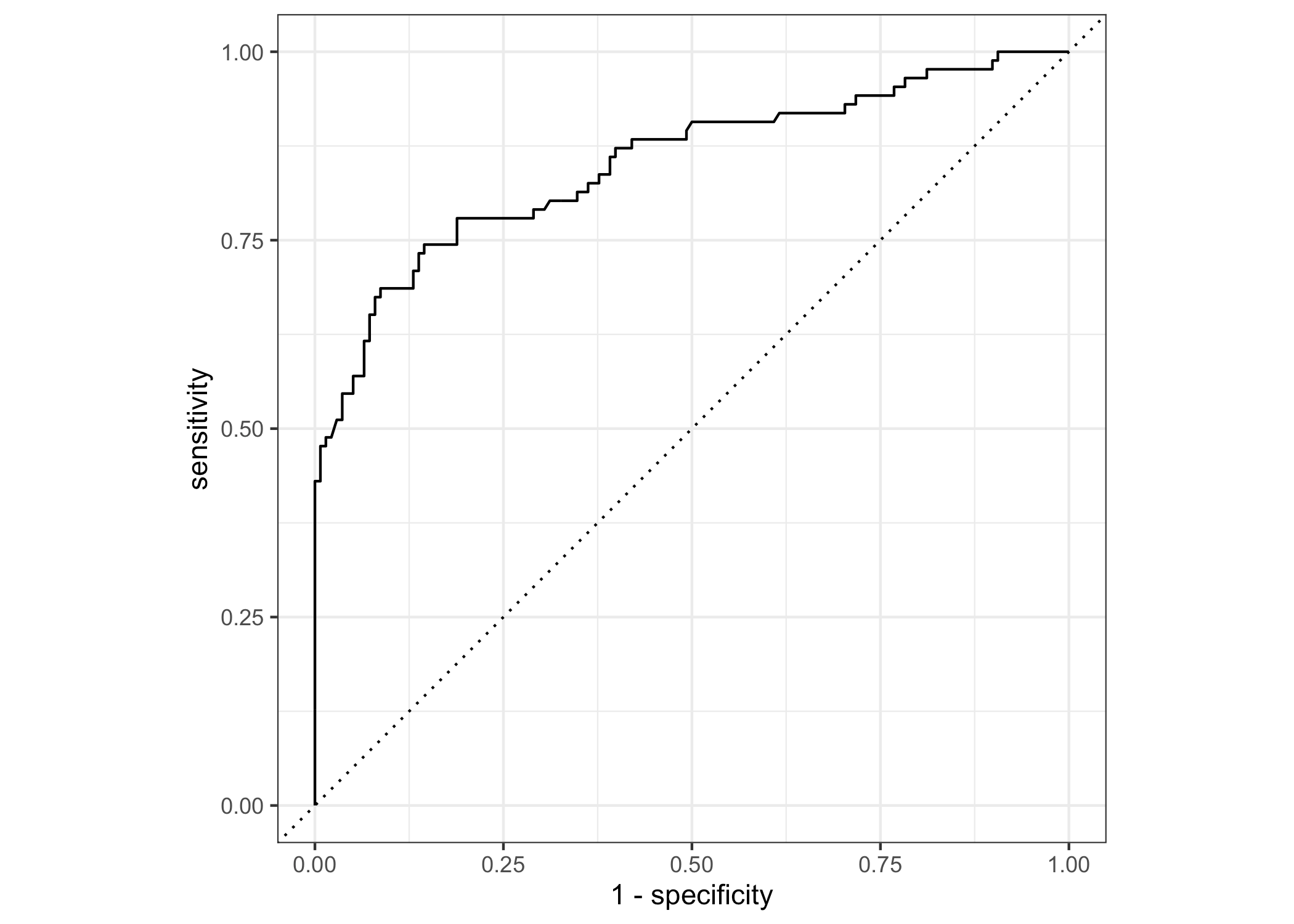Now we’re ready to predict survival for the holdout dataset and submit to Kaggle.

```logistic_wf %>%
fit(dataset) %>%
augment(holdout) %>%
select(PassengerId, Survived = .pred_class) %>%
mutate(Survived = if_else(Survived == "yes", 1, 0)) %>%
write_csv("output/titanic/logistic.csv")
```

I got and accuracy of 0.76076. Oldies but goodies!

# Neural networks

We go on with neural networks.

## Tuning

Set up defaults.

```mset <- metric_set(accuracy) # metric is accuracy
control <- control_grid(save_workflow = TRUE,
save_pred = TRUE,
extract = extract_model) # grid for tuning
```

First a recipe.

```nn_rec <- recipe(survived ~ ., data = train) %>%
step_impute_median(all_numeric()) %>% # replace missing value by median
step_normalize(all_numeric_predictors()) %>%
step_dummy(all_nominal_predictors()) # all factors var are split into binary terms (factor disj coding)
```

Then specify a neural network.

```nn_model <- mlp(epochs = tune(),
hidden_units = tune(),
dropout = tune()) %>% # param to be tuned
set_mode("classification") %>% # binary response var
set_engine("keras", verbose = 0)
```

Now set our workflow.

```nn_wf <-
workflow() %>%
```

Use cross-validation to evaluate our model with different param config.

```nn_tune <- nn_wf %>%
tune_race_anova(
train_5fold,
grid = 30,
param_info = nn_model %>% parameters(),
metrics = metric_set(accuracy),
control = control_race(verbose_elim = TRUE))
```

Visualize the results.

```autoplot(nn_tune)
```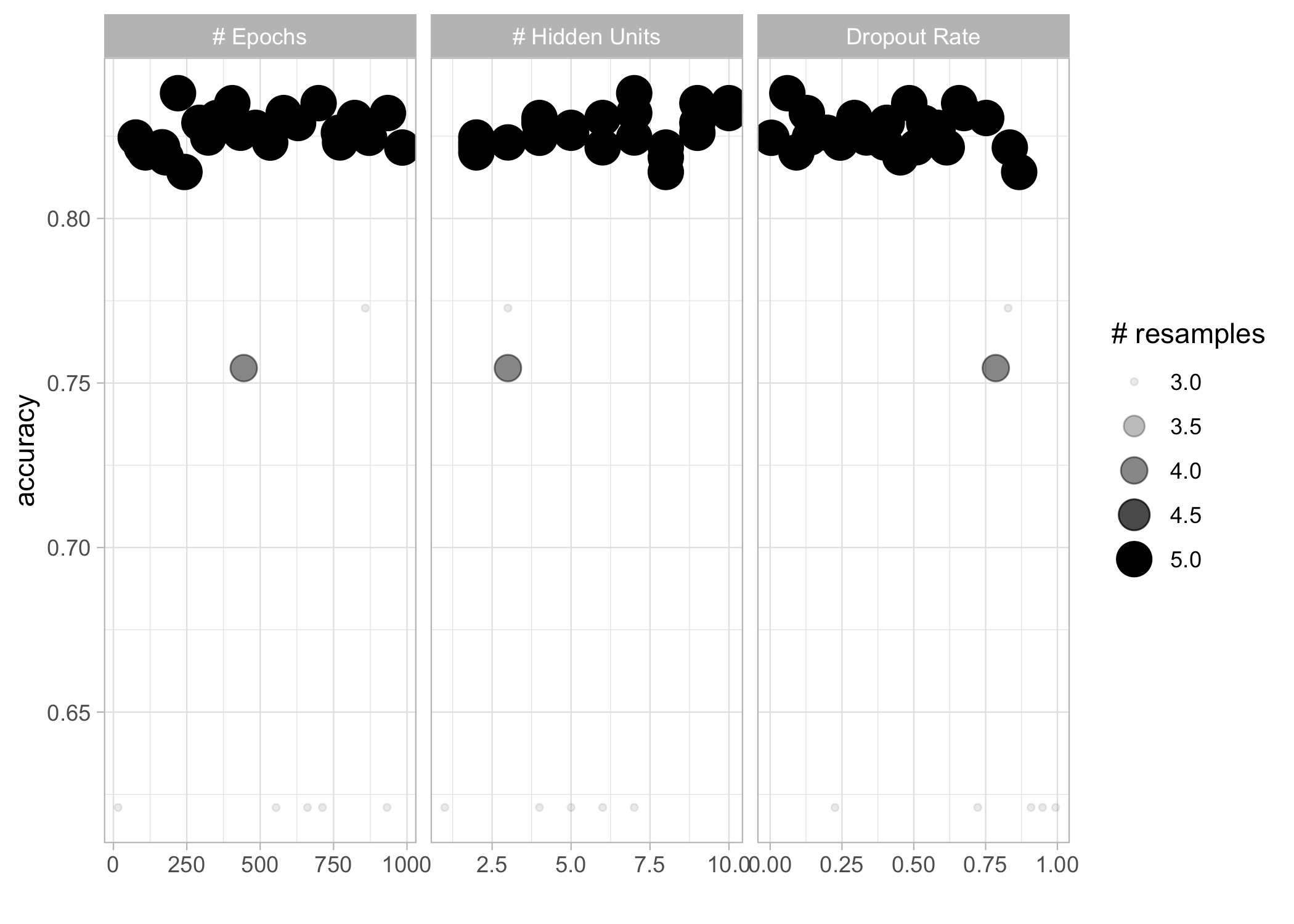Collect metrics.

```nn_tune %>%
collect_metrics() %>%
arrange(desc(mean))
## # A tibble: 30 × 9
## hidden_units dropout epochs .metric .estimator mean n std_err .config
## <int> <dbl> <int> <chr> <chr> <dbl> <int> <dbl> <chr>
## 1 10 0.484 405 accuracy binary 0.838 5 0.0150 Preprocessor1_Model…
## 2 7 0.0597 220 accuracy binary 0.834 5 0.00881 Preprocessor1_Model…
## 3 4 0.536 629 accuracy binary 0.834 5 0.0154 Preprocessor1_Model…
## 4 9 0.198 768 accuracy binary 0.832 5 0.0146 Preprocessor1_Model…
## 5 6 0.752 822 accuracy binary 0.832 5 0.0112 Preprocessor1_Model…
## 6 9 0.406 293 accuracy binary 0.831 5 0.0145 Preprocessor1_Model…
## 7 4 0.00445 871 accuracy binary 0.831 5 0.0150 Preprocessor1_Model…
## 8 4 0.293 353 accuracy binary 0.831 5 0.0117 Preprocessor1_Model…
## 9 10 0.675 935 accuracy binary 0.831 5 0.0141 Preprocessor1_Model…
## 10 7 0.128 580 accuracy binary 0.831 5 0.0151 Preprocessor1_Model…
## # … with 20 more rows
```

## Fit model

Use best config to fit model to training data.

```nn_fit <- nn_wf %>%
finalize_workflow(select_best(nn_tune)) %>%
fit(train)
```

Check out accuracy on testing dataset to see if we overfitted.

```nn_fit %>%
augment(test, type.predict = "response") %>%
accuracy(survived, .pred_class)
## # A tibble: 1 × 3
## .metric .estimator .estimate
## <chr> <chr> <dbl>
## 1 accuracy binary 0.817
```

## Make predictions

Now we’re ready to predict survival for the holdout dataset and submit to Kaggle.

```nn_wf %>%
finalize_workflow(select_best(nn_tune)) %>%
fit(train) %>%
augment(holdout) %>%
select(PassengerId, Survived = .pred_class) %>%
mutate(Survived = if_else(Survived == "yes", 1, 0)) %>%
write_csv("output/titanic/nn.csv")
```

I got and accuracy of 0.78708. My best score so far.

# Support vector machines

We go on with support vector machines.

## Tuning

Set up defaults.

```mset <- metric_set(accuracy) # metric is accuracy
control <- control_grid(save_workflow = TRUE,
save_pred = TRUE,
extract = extract_model) # grid for tuning
```

First a recipe.

```svm_rec <- recipe(survived ~ ., data = train) %>%
step_impute_median(all_numeric()) %>% # replace missing value by median
# remove any zero variance predictors
step_zv(all_predictors()) %>%
# remove any linear combinations
step_lincomb(all_numeric()) %>%
step_dummy(all_nominal_predictors()) # all factors var are split into binary terms (factor disj coding)
```

Then specify a svm.

```svm_model <- svm_rbf(cost = tune(),
rbf_sigma = tune()) %>% # param to be tuned
set_mode("classification") %>% # binary response var
set_engine("kernlab")
```

Now set our workflow.

```svm_wf <-
workflow() %>%
```

Use cross-validation to evaluate our model with different param config.

```svm_tune <- svm_wf %>%
tune_race_anova(
train_5fold,
grid = 30,
param_info = svm_model %>% parameters(),
metrics = metric_set(accuracy),
control = control_race(verbose_elim = TRUE))
```

Visualize the results.

```autoplot(svm_tune)
```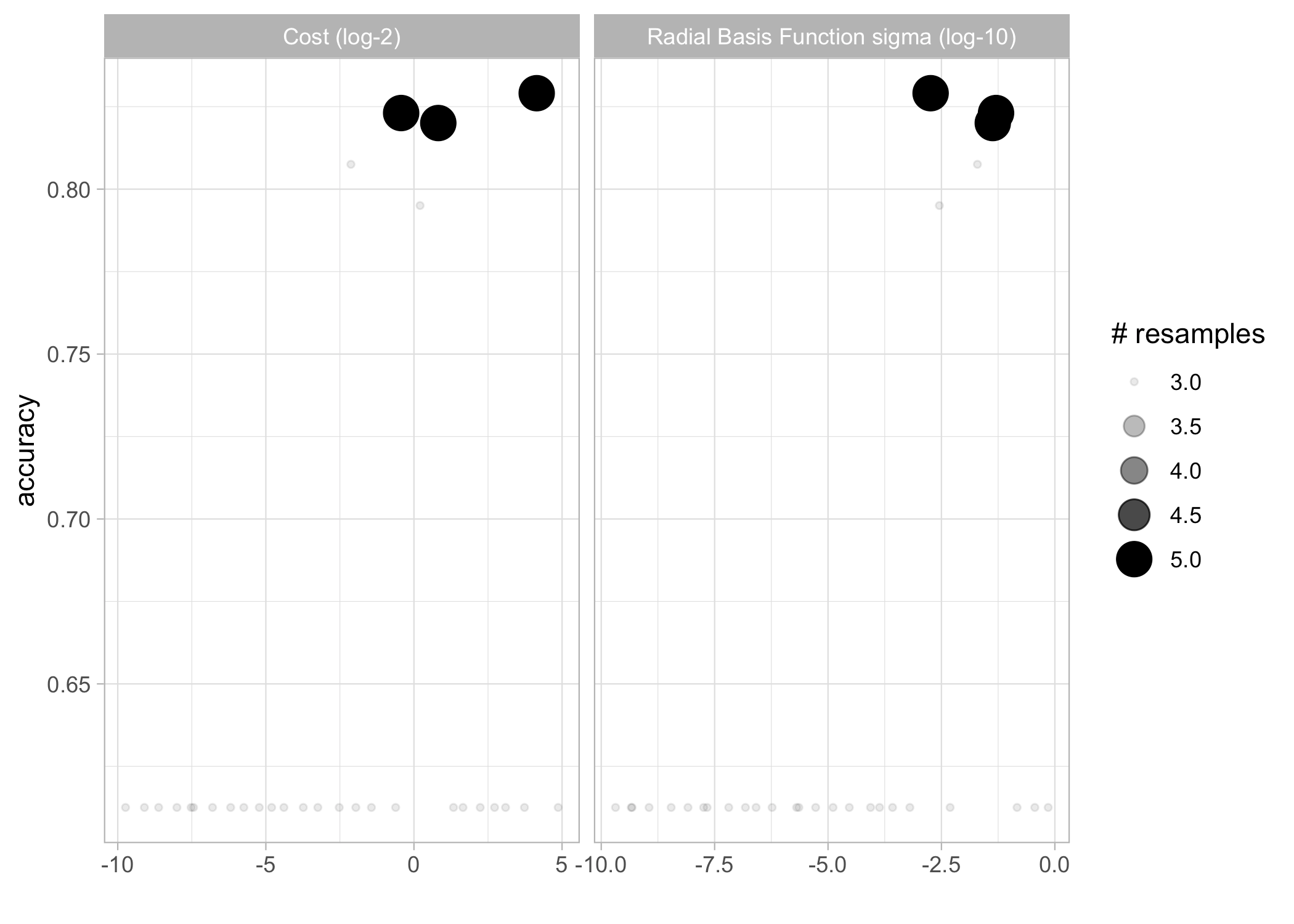Collect metrics.

```svm_tune %>%
collect_metrics() %>%
arrange(desc(mean))
## # A tibble: 30 × 8
## cost rbf_sigma .metric .estimator mean n std_err .config
## <dbl> <dbl> <chr> <chr> <dbl> <int> <dbl> <chr>
## 1 17.7 0.00183 accuracy binary 0.829 5 0.0101 Preprocessor1_Model05
## 2 0.741 0.0506 accuracy binary 0.823 5 0.0129 Preprocessor1_Model14
## 3 1.77 0.0429 accuracy binary 0.820 5 0.0143 Preprocessor1_Model08
## 4 0.229 0.0197 accuracy binary 0.808 3 0.00626 Preprocessor1_Model23
## 5 1.15 0.00285 accuracy binary 0.795 3 0.00867 Preprocessor1_Model28
## 6 0.00182 0.00000203 accuracy binary 0.613 3 0.0171 Preprocessor1_Model01
## 7 0.0477 0.714 accuracy binary 0.613 3 0.0171 Preprocessor1_Model02
## 8 6.60 0.0000294 accuracy binary 0.613 3 0.0171 Preprocessor1_Model03
## 9 0.00254 0.000636 accuracy binary 0.613 3 0.0171 Preprocessor1_Model04
## 10 0.00544 0.0000000647 accuracy binary 0.613 3 0.0171 Preprocessor1_Model06
## # … with 20 more rows
```

## Fit model

Use best config to fit model to training data.

```svm_fit <- svm_wf %>%
finalize_workflow(select_best(svm_tune)) %>%
fit(train)
```

Check out accuracy on testing dataset to see if we overfitted.

```svm_fit %>%
augment(test, type.predict = "response") %>%
accuracy(survived, .pred_class)
## # A tibble: 1 × 3
## .metric .estimator .estimate
## <chr> <chr> <dbl>
## 1 accuracy binary 0.826
```

## Make predictions

Now we’re ready to predict survival for the holdout dataset and submit to Kaggle.

```svm_wf %>%
finalize_workflow(select_best(svm_tune)) %>%
fit(dataset) %>%
augment(holdout) %>%
select(PassengerId, Survived = .pred_class) %>%
mutate(Survived = if_else(Survived == "yes", 1, 0)) %>%
write_csv("output/titanic/svm.csv")
```

I got and accuracy of 0.77511.

# Decision trees

We go on with decision trees.

## Tuning

Set up defaults.

```mset <- metric_set(accuracy) # metric is accuracy
control <- control_grid(save_workflow = TRUE,
save_pred = TRUE,
extract = extract_model) # grid for tuning
```

First a recipe.

```dt_rec <- recipe(survived ~ ., data = train) %>%
step_impute_median(all_numeric()) %>% # replace missing value by median
step_zv(all_predictors()) %>%
step_dummy(all_nominal_predictors()) # all factors var are split into binary terms (factor disj coding)
```

Then specify a decision tree model.

```library(baguette)
dt_model <- bag_tree(cost_complexity = tune(),
tree_depth = tune(),
min_n = tune()) %>% # param to be tuned
set_engine("rpart", times = 25) %>% # nb bootstraps
set_mode("classification") # binary response var
```

Now set our workflow.

```dt_wf <-
workflow() %>%
```

Use cross-validation to evaluate our model with different param config.

```dt_tune <- dt_wf %>%
tune_race_anova(
train_5fold,
grid = 30,
param_info = dt_model %>% parameters(),
metrics = metric_set(accuracy),
control = control_race(verbose_elim = TRUE))
```

Visualize the results.

```autoplot(dt_tune)
```Collect metrics.

```dt_tune %>%
collect_metrics() %>%
arrange(desc(mean))
## # A tibble: 30 × 9
## cost_complexity tree_depth min_n .metric .estimator mean n std_err .config
## <dbl> <int> <int> <chr> <chr> <dbl> <int> <dbl> <chr>
## 1 3.02e- 7 13 7 accuracy binary 0.832 5 0.0130 Preprocessor1_…
## 2 3.43e- 3 14 4 accuracy binary 0.829 5 0.0145 Preprocessor1_…
## 3 5.75e- 5 5 7 accuracy binary 0.828 5 0.0126 Preprocessor1_…
## 4 2.13e- 6 14 5 accuracy binary 0.828 5 0.0108 Preprocessor1_…
## 5 1.34e- 5 5 35 accuracy binary 0.823 5 0.00654 Preprocessor1_…
## 6 4.47e- 5 13 10 accuracy binary 0.823 5 0.0120 Preprocessor1_…
## 7 1.42e- 2 4 25 accuracy binary 0.822 5 0.0121 Preprocessor1_…
## 8 4.54e-10 10 36 accuracy binary 0.822 5 0.0147 Preprocessor1_…
## 9 8.10e- 8 11 32 accuracy binary 0.822 5 0.0143 Preprocessor1_…
## 10 3.43e- 4 11 21 accuracy binary 0.820 5 0.0207 Preprocessor1_…
## # … with 20 more rows
```

## Fit model

Use best config to fit model to training data.

```dt_fit <- dt_wf %>%
finalize_workflow(select_best(dt_tune)) %>%
fit(train)
```

Check out accuracy on testing dataset to see if we overfitted.

```dt_fit %>%
augment(test, type.predict = "response") %>%
accuracy(survived, .pred_class)
## # A tibble: 1 × 3
## .metric .estimator .estimate
## <chr> <chr> <dbl>
## 1 accuracy binary 0.808
```

## Make predictions

Now we’re ready to predict survival for the holdout dataset and submit to Kaggle.

```dt_wf %>%
finalize_workflow(select_best(dt_tune)) %>%
fit(dataset) %>%
augment(holdout) %>%
select(PassengerId, Survived = .pred_class) %>%
mutate(Survived = if_else(Survived == "yes", 1, 0)) %>%
write_csv("output/titanic/dt.csv")
```

I got and accuracy of 0.76794.

# Stacked ensemble modelling

Let’s do some ensemble modelling with all algo but logistic and catboost. Tune again with a probability-based metric. Start with xgboost.

```library(finetune)
library(stacks)
# xgboost
xg_rec <- recipe(survived ~ ., data = train) %>%
step_impute_median(all_numeric()) %>% # replace missing value by median
step_dummy(all_nominal_predictors()) # all factors var are split into binary terms (factor disj coding)
xg_model <- boost_tree(mode = "classification", # binary response
trees = tune(),
mtry = tune(),
tree_depth = tune(),
learn_rate = tune(),
loss_reduction = tune(),
min_n = tune()) # parameters to be tuned
xg_wf <-
workflow() %>%
xg_grid <- grid_latin_hypercube(
trees(),
finalize(mtry(), train),
tree_depth(),
learn_rate(),
loss_reduction(),
min_n(),
size = 30)
xg_tune <- xg_wf %>%
tune_grid(
resamples = train_5fold,
grid = xg_grid,
metrics = metric_set(roc_auc),
control = control_stack_grid())
```

Then random forests.

```# random forest
rf_rec <- recipe(survived ~ ., data = train) %>%
step_impute_median(all_numeric()) %>%
step_dummy(all_nominal_predictors())
rf_model <- rand_forest(mode = "classification", # binary response
engine = "ranger", # by default
mtry = tune(),
trees = tune(),
min_n = tune()) # parameters to be tuned
rf_wf <-
workflow() %>%
rf_grid <- grid_latin_hypercube(
finalize(mtry(), train),
trees(),
min_n(),
size = 30)
rf_tune <- rf_wf %>%
tune_grid(
resamples = train_5fold,
grid = rf_grid,
metrics = metric_set(roc_auc),
control = control_stack_grid())
```

Regularisation methods (between ridge and lasso).

```# regularization methods
en_rec <- recipe(survived ~ ., data = train) %>%
step_impute_median(all_numeric()) %>% # replace missing value by median
step_normalize(all_numeric_predictors()) %>% # normalize
step_dummy(all_nominal_predictors())
en_model <- logistic_reg(penalty = tune(),
mixture = tune()) %>% # param to be tuned
set_engine("glmnet") %>% # elastic net
set_mode("classification") # binary response
en_wf <-
workflow() %>%
en_grid <- grid_latin_hypercube(
penalty(),
mixture(),
size = 30)
en_tune <- en_wf %>%
tune_grid(
resamples = train_5fold,
grid = en_grid,
metrics = metric_set(roc_auc),
control = control_stack_grid())
```

Neural networks (takes time, so pick only a few values for illustration purpose).

```# neural networks
nn_rec <- recipe(survived ~ ., data = train) %>%
step_impute_median(all_numeric()) %>% # replace missing value by median
step_normalize(all_numeric_predictors()) %>%
step_dummy(all_nominal_predictors())
nn_model <- mlp(epochs = tune(),
hidden_units = 2,
dropout = tune()) %>% # param to be tuned
set_mode("classification") %>% # binary response var
set_engine("keras", verbose = 0)
nn_wf <-
workflow() %>%
# nn_grid <- grid_latin_hypercube(
# epochs(),
# hidden_units(),
# dropout(),
# size = 10)
nn_tune <- nn_wf %>%
tune_grid(
resamples = train_5fold,
grid = crossing(dropout = c(0.1, 0.2), epochs = c(250, 500, 1000)), # nn_grid
metrics = metric_set(roc_auc),
control = control_stack_grid())
#autoplot(nn_tune)
```

Support vector machines.

```# support vector machines
svm_rec <- recipe(survived ~ ., data = train) %>%
step_impute_median(all_numeric()) %>%
step_normalize(all_numeric_predictors()) %>%
step_dummy(all_nominal_predictors())
svm_model <- svm_rbf(cost = tune(),
rbf_sigma = tune()) %>% # param to be tuned
set_mode("classification") %>% # binary response var
set_engine("kernlab")
svm_wf <-
workflow() %>%
svm_grid <- grid_latin_hypercube(
cost(),
rbf_sigma(),
size = 30)
svm_tune <- svm_wf %>%
tune_grid(
resamples = train_5fold,
grid = svm_grid,
metrics = metric_set(roc_auc),
control = control_stack_grid())
```

Last, decision trees.

```# decision trees
dt_rec <- recipe(survived ~ ., data = train) %>%
step_impute_median(all_numeric()) %>%
step_zv(all_predictors()) %>%
step_dummy(all_nominal_predictors())
library(baguette)
dt_model <- bag_tree(cost_complexity = tune(),
tree_depth = tune(),
min_n = tune()) %>% # param to be tuned
set_engine("rpart", times = 25) %>% # nb bootstraps
set_mode("classification") # binary response var
dt_wf <-
workflow() %>%
dt_grid <- grid_latin_hypercube(
cost_complexity(),
tree_depth(),
min_n(),
size = 30)
dt_tune <- dt_wf %>%
tune_grid(
resamples = train_5fold,
grid = dt_grid,
metrics = metric_set(roc_auc),
control = control_stack_grid())
```

Get best config.

```xg_best <- xg_tune %>% filter_parameters(parameters = select_best(xg_tune))
rf_best <- rf_tune %>% filter_parameters(parameters = select_best(rf_tune))
en_best <- en_tune %>% filter_parameters(parameters = select_best(en_tune))
nn_best <- nn_tune %>% filter_parameters(parameters = select_best(nn_tune))
svm_best <- svm_tune %>% filter_parameters(parameters = select_best(svm_tune))
dt_best <- dt_tune %>% filter_parameters(parameters = select_best(dt_tune))
```

Do the stacked ensemble modelling.

Pile all models together.

```blended <- stacks() %>% # initialize
blended
## # A data stack with 6 model definitions and 6 candidate members:
## # en_best: 1 model configuration
## # xg_best: 1 model configuration
## # rf_best: 1 model configuration
## # nn_best: 1 model configuration
## # svm_best: 1 model configuration
## # dt_best: 1 model configuration
## # Outcome: survived (factor)
```

Fit regularized model.

```blended_fit <- blended %>%
blend_predictions() # fit regularized model
```

Visualise penalized model. Note that neural networks are dropped, despite achieving best score when used in isolation. I’ll have to dig into that.

```autoplot(blended_fit)
``````autoplot(blended_fit, type = "members")
```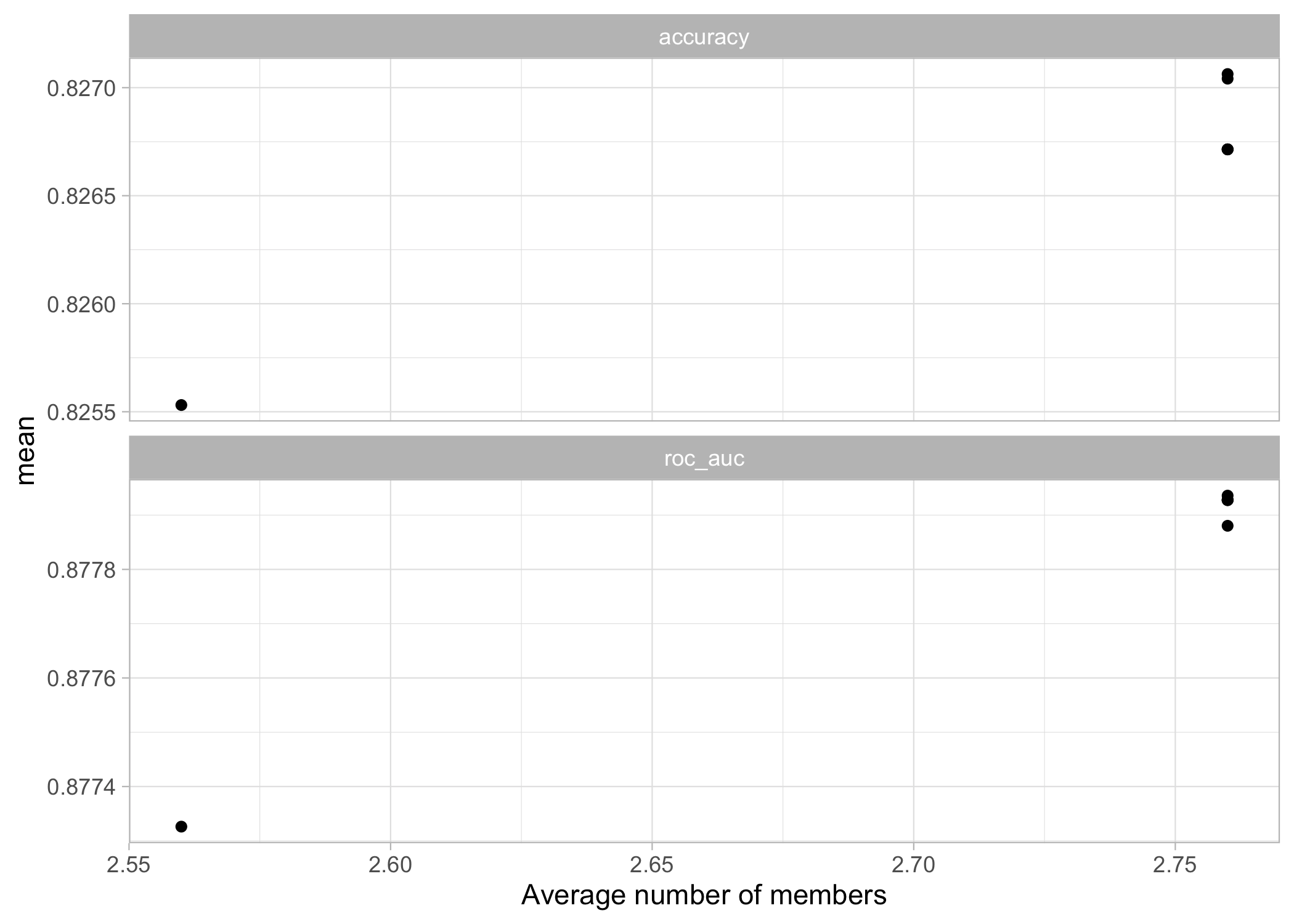```autoplot(blended_fit, type = "weights")
```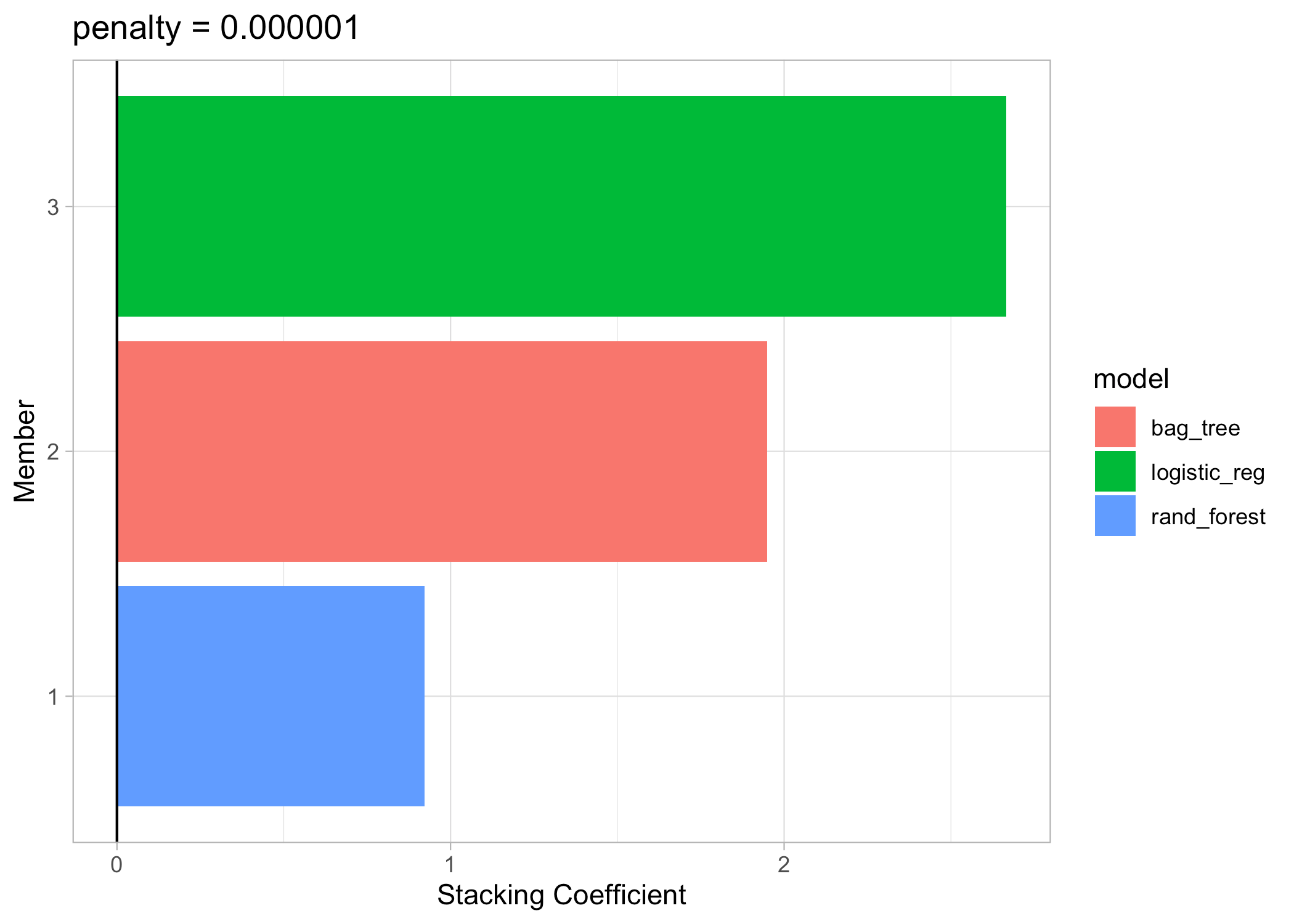Fit candidate members with non-zero stacking coef with full training dataset.

```blended_regularized <- blended_fit %>%
fit_members()
blended_regularized
## # A tibble: 3 × 3
## member type weight
## <chr> <chr> <dbl>
## 1 .pred_no_en_best_1_12 logistic_reg 2.67
## 2 .pred_no_dt_best_1_11 bag_tree 1.95
## 3 .pred_no_rf_best_1_05 rand_forest 0.922
```

Perf on testing dataset?

```test %>%
bind_cols(predict(blended_regularized, .)) %>%
accuracy(survived, .pred_class)
## # A tibble: 1 × 3
## .metric .estimator .estimate
## <chr> <chr> <dbl>
## 1 accuracy binary 0.826
```

Now predict.

```holdout %>%
bind_cols(predict(blended_regularized, .)) %>%
select(PassengerId, Survived = .pred_class) %>%
mutate(Survived = if_else(Survived == "yes", 1, 0)) %>%
write_csv("output/titanic/stacked.csv")
```

I got an 0.76076 accuracy.

# Conclusions

I covered several ML algorithms and logistic regression with the awesome `tidymodels` metapackage in `R`. My scores at predicting Titanic survivors were ok I guess. Some folks on Kaggle got a perfect accuracy, so there is always room for improvement. Maybe better tuning, better features (or predictors) or other algorithms would increase accuracy. Of course, I forgot to use `set.seed()` so results are not exactly reproducible.

1. This post was also published on https://www.r-bloggers.com. ↩︎